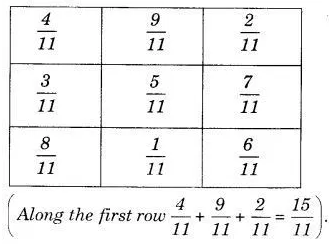# In a “magic square”, the sum of the numbers in each row,

In a “magic square”, the sum of the numbers in each row, in each column and along the diagonals is the same. Is this a magic square?